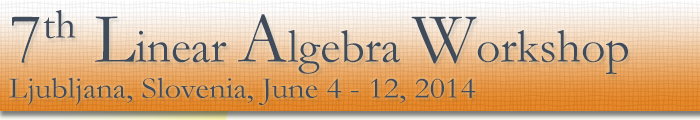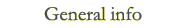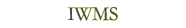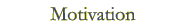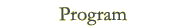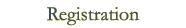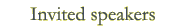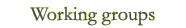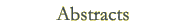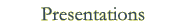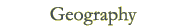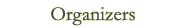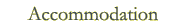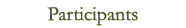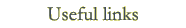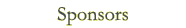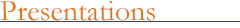Invited ILAS lecture Alexander Guterman: Pólya permanent problem: 100 years after Invited talks John Holbrook: Hawaiian volcanoes and complete polynomial bounds Thomas Laffey: Patterned matrices with explicit trace vector and some consequences Leo Livshits: (Exactly) Paratransitive algebras of linear transformations Laurent Marcoux: Extending semigroups of partial isometries Mitja Mastnak: On semitransitivity Vladimir Müler: On distributionally irregular vectors Heydar Radjavi: Simultaneous versions of Wielandt's positivity theorem Leiba Rodman: Numerical ranges of quaternion matrices Vladimir Troitsky: Multinorms and Banach lattices Contributed talks Anita Buckley: Simultaneously self-adjoint sets of matrices Mikhail Budrevich: Matrix convertibility over finite field György Gehėr: An elementary proof of Wigner's theorem on quantum mechanical symmetry transformations Marko Kandić: Complete decomposability of positive compact operators with positive commutators Endre Makai: Local and global liftings of analytic families of idempotents in Banach algebras Olga Markova: On the length of matrix algebras Bojan Mohar: On median eigenvalues of graphs Polona Oblak: The total graphs of finite commutative semirings Lina Oliveira: The Erdos density theorem revisited Aljoša Peperko: On the spectrum and the spectral mapping theorem in max algebra Al Sethuraman: Skew-hermitian skew-commuting matrices and fast-decodability of space-time codes Helena Šmigoc: Patterns of symmetric matrices that allow all the eigenvalue multiplicities to be even Špela Špenko: Identities of matrix algebras Stephan Weis: Open linear maps and geometry of the numerical range Presentations at International Workshop on Matrices and Statistics